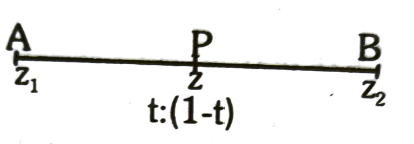Algebra of complex numbers
Question

# Let z1 and z2, be two distinct complex numbers and let  for some real number t with 0 <  t < 1. if arg (w) denotes the principal argument of a non-zero complex number , then

Moderate
Solution

## Given $\mathrm{z}=\frac{\left(1-\mathrm{t}\right){\mathrm{z}}_{1}+{\mathrm{tz}}_{2}}{\left(1-\mathrm{t}\right)+\mathrm{t}}$Clearly, z divides z, and z, in the ratio of t :(1 - t), 0<t<1and $\mathrm{arg}\left(\mathrm{z}-{\mathrm{z}}_{1}\right)=\mathrm{arg}\left({\mathrm{z}}_{2}-\mathrm{z}\right)=\mathrm{arg}\left({\mathrm{z}}_{2}-{\mathrm{z}}_{1}\right)$Also ,$\mathrm{arg}\left(\mathrm{z}-{\mathrm{z}}_{1}\right)=\mathrm{arg}\left({\mathrm{z}}_{2}-{\mathrm{z}}_{1}\right)$$⇒\mathrm{arg}\left(\frac{\mathrm{z}-{\mathrm{z}}_{1}}{{\mathrm{z}}_{2}-{\mathrm{z}}_{1}}\right)=0$$\therefore \frac{z-{z}_{1}}{{z}_{2}-{z}_{1}}$ is purely real.

Get Instant Solutions